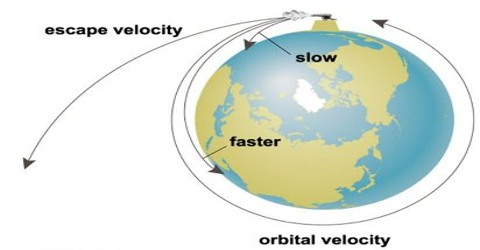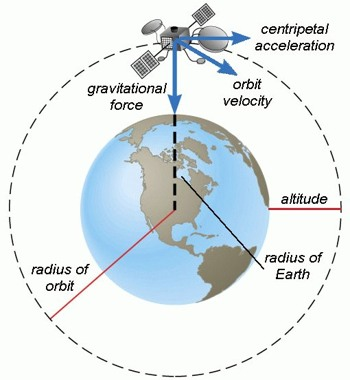Physics

# Orbital VelocityOrbital velocity

Objects that travel in uniform circular motion around the Earth are said to be “in orbit”. The velocity of this orbit depends on the distance from the object to the centre of the Earth. Orbital velocity of a satellite revolving around the earth at a height h from the earth is v = √GM / (R + h), where R and M are respectively radius and mass of the earth. So it is seen that orbital velocity is not dependent on the mass of the satellite. That means two satellites will revolve at the same speed.Information: If an object is thrown upward from the surface of the earth with velocity different phenomena of the object may happen by the attractive force of the earth, viz:

(1) If v2 < vE2/2, i.e., if the velocity of ejection is less than 7.88 kms-1, then it will revolve round the earth in elliptical orbit and finally will come back in the earth.

(2) If v2 = vE2/2, i.e., the velocity of get-ton lb equal to 7.88 kms-1, then the object will rotate round the earth and will be a satellite like a moon.

(3) If v2 > vE2/2, but vE2 i.e., the velocity of ejection is between 7.88 kms-1 and 11.2 kms-2, then the object will continue to rotate round the earth in elliptical orbit keeping the earth at one focus

(4) If v = vE, i.e., the velocity of election is 11.2 kms-1 i.e., equal to the escape velocity, the object will leave the surface of the earth in the parabolic path and will go outside the influence of gravitation force of attraction.

(5) If v > vE, i.e., velocity of election is greater than the escape velocity, then in parabolic path the object will leave the earth and will not come back in the earth.